### Home > CALC > Chapter 5 > Lesson 5.2.1 > Problem5-55

5-55.
1. Draw the graph of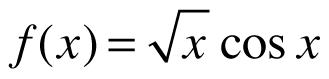for 0 ≤ x ≤ 3. Homework Help ✎

1. Find f ′(x).

2. Graph f ′(x) using your graphing calculator. Then, use this graph to find the x-value of the local maximum of f(x).

3. Likewise, use a graph of f ″(x) to find the point of inflection ofbetween 0 ≤ x ≤ 3.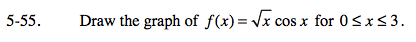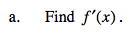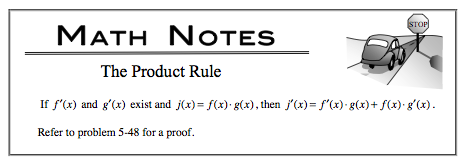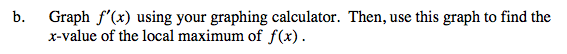The location of a local maximum on f(x) can be found where f '(x) = 0 AND changes from positive values to negative values.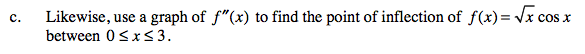A zero on the second derivative that crosses the x-axis (or changes sign) indicates a point of inflection on the original function.

Use the eTool below to help solve the problem.
Click the link at right for the full version of the eTool: Calc 5-55 HW eTool